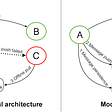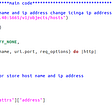# Activate Fountain

`There is a one-dimensional garden on the x-axis. The garden starts at the point 0 and ends at the point n. (i.e The length of the garden is n).There are n + 1 taps located at points [0, 1, ..., n] in the garden.Given an integer n and an integer array ranges of length n + 1 where ranges[i] (0-indexed) means the i-th tap can water the area [i - ranges[i], i + ranges[i]] if it was open.Return the minimum number of taps that should be open to water the whole garden, If the garden cannot be watered return -1.`
`for i in range(len(ranges)):    for j in range(max(0, i - ranges[i]), min(len(ranges)-1, i - ranges[i])):        if(i != j and ranges[j] != -1 and max(0, i - ranges[i]) <= max(0, j- ranges[j]) and min(len(ranges)-1, i - ranges[i]) >= min(len(ranges)-1, i - ranges[j]):            ranges[j] = -1count = 0for i in range(len(ranges)):    if(ranges[i] > -1):        count += 1return count`
`for i in range(len(ranges)):    for j in range(max(0, i - ranges[i]), min(len(ranges)-1, i - ranges[i])):        if(i != j and ranges[j] != -1 and max(0, i - ranges[i]) <= max(0, j- ranges[j]) and min(len(ranges)-1, i - ranges[i]) >= min(len(ranges)-1, i - ranges[j])):            ranges[j] = -1left = falseright = falsefor i in range(len(ranges)):    for j in range(max(0, i - ranges[i]), min(len(ranges)-1, i - ranges[i])):        if(i != j and ranges[j] != -1 and max(0, i - ranges[i]) <= max(0, j- ranges[j])):            left = true        if(i != j and ranges[j] != -1 and min(len(ranges)-1, i - ranges[i]) >= min(len(ranges)-1, i - ranges[j])):            right = true    if(left and right):        range[i] = -1count = 0for i in range(len(ranges)):    if(ranges[i] > -1):        count += 1return count`
`def minTaps(self, n: int, ranges: List[int]) -> int:    new_list = a =  * (n + 1)    for i in range(n + 1):         left = max(0, i - ranges[i])         right = min(n, i + ranges[i])         new_list[left] = max(new_list[left], right - left)    start = end = count = 0    while(end < n):        count += 1        start, end = end, max(i + new_list[i] for i in range(start, end + 1))        if(start == end):            return -1return count`

--

--

--

## More from Aidanmc

Love podcasts or audiobooks? Learn on the go with our new app.

## My Annual Planning Process## Create a WordPress using Docker on Alibaba Cloud## An Easy Way of Deploying Helm Charts on Red Hat OpenShift## Implementation of Message Push and Storage Architectures of Modern IM Systems## The Most Promising Fields for Programming in the Future## Sprintnotes 10: A sprint of firsts## Auto healing monitoring alert system : part 03## Building an image classifier from scratch using Convolutional Neural Networks.## Can we difference artists by the colors they use?## Next Word Prediction using Long Short Term Memory (LSTM)